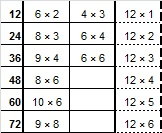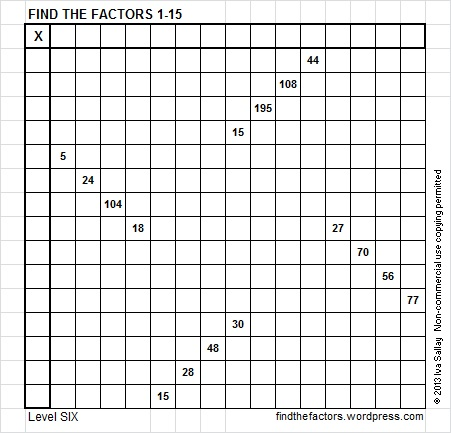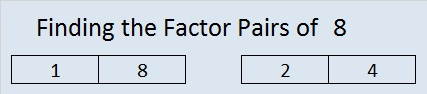# 8 What Happens When Puzzle Dimensions Change?

My daughter-in-law, Julayne, is brilliant. She earned a master’s degree in mathematics and teaches at the college level. She is also an expert at solving the Hungarian puzzle known as a Rubik’s cube. Since she can easily solve a 3 x 3 x 3 cube, she decided to conquer 4 x 4 x 4, 5 x 5 x 5, and 6 x 6 x 6 cubes. She has even been able to solve the 7 x 7 x 7 cube. Amazing! If you hand her a physical cube in any of those dimensions, she will be able to solve it. She can even easily solve a virtual Rubik’s cube.

When I showed her the Find the Factors puzzles, she naturally asked if I could make one that used factors all the way to fifteen.

Do the dimensions of the puzzle matter? The puzzles usually ask you to find the factors from 1 to 10 or 1 to 12. What if we had a Find the Factors 1-5 puzzle?This puzzle is so easy to solve that most people will not think it worth their time. The number combinations are so few that it should never prove to be very challenging. If you solve this puzzle, you may find it hard to believe that the numbers were chosen at random, but they really were. You probably will agree with me that 5 factors are too few. If we made puzzles with 6 factors, then 7, 8, or 9, they would all be more difficult than a 5-factor puzzle, but a 10-factor puzzle seems like the best place to start. Most everyone is expected to know the times table up to 10 x 10 = 100. Many people were also taught the multiplication facts up to 12 x 12 = 144.

A coworker once mentioned to me that he noticed that puzzles with 12 factors are much more difficult to solve than ones with 10 factors. Whether the factors are prime or composite numbers is only part of what makes a puzzle easy or difficult. For example, even though 2, 3, and 5 are prime numbers, they don’t make a puzzle easier because they have multiples that can also be factors in the puzzle. Prime numbers 7 and 11 help make a puzzle easier, so a Find the Factors 1-11 would actually be easier than a 10-factor puzzle. Adding 12 complicates the puzzle significantly because the following multiples of 12 have other factors that also could be needed to solve the puzzle.Thirteen, another prime number, also makes the puzzle a little easier. My brother, Andy, will have his 65 birthday soon. I made him a Find the Factors 1-13 puzzle because I wanted to include the number 65 which is 13 x 5. Most people only know a few of the 13 multiplication facts like 4 suits x 13 ranks = 52 playing cards, but if you solve this puzzle, you will still probably find it easier than a 1-12 factor puzzle.Adding 14 as a possible factor takes away the advantages of prime number 7, so a 14-factor puzzle would be more difficult. Also, most people have not memorized the first 14 multiples of 14. Making or solving a 15-factor puzzle makes the multiples of 3 and 5 become even more complicated clues. Of course, most people don’t recall the first 15 multiples of 15 either. The many possible factors for the clues make it more difficult to create a puzzle that has only one solution. Try to solve this Find the Factors 1-15 puzzle. It expects you to know the two factors of 195 that are both between 1 and 15. Also, the common factor of 15 and 30 could be 3, 5, or 15. This puzzle can still be solved using logic only, but it will be more challenging than puzzles of smaller dimensions.You can cut and paste the puzzle into a document and make it any size you wish or you can open 10 Factors 2013-12-02 to view it along with the following puzzles.

• 8 is a composite number.
• Prime factorization: 8 = 2 × 2 × 2, which can be written 8 = 2³
• The exponent in the prime factorization is 3. Adding one, we get (3 + 1) = 4. Therefore 8 has exactly 4 factors.
• Factors of 8: 1, 2, 4, 8
• Factor pairs: 8 = 1 × 8 or 2 × 4
• Taking the factor pair with the largest square number factor, we get √8 = (√4)(√2) = 2√2 ≈ 2.828
• 8 is a perfect cube.When 8 is a clue in the FIND THE FACTORS puzzles, use either 1 x 8 or 2 x 4.

Here’s a cool tweet about the number 8: